# Catch up on the latest AI articles# Dropout Layers, Not Weights Or Nodes! "LayerDrop" Proposal

3 main points
✔️ Transformer has a large number of parameters and requires a huge amount of computation
✔️ We propose LayerDrop, which is a new layer dropout as model compression
✔️ Extract models with varying number of layers without changing the original performance

written by Angela FanEdouard GraveArmand Joulin
(Submitted on 25 Sep 2019)

Subjects: Machine Learning (cs.LG); Computation and Language (cs.CL); Machine Learning (stat.ML)code：## first of all

Transformer is a key architecture in natural language processing such as machine translation, where each layer of the transformer contains millions of parameters and requires a huge amount of computation for both learning and inference.

Model compression is the process of extracting a smaller (less computationally expensive) model while maintaining the performance of a larger model such as a Transformer. There are two main methods for model compression: the first is Distillation, which uses the output of the original model as supervised data to train a smaller model, and the second is Pruning, which reduces the number of parameters by removing nodes and weights from the model. Pruning is a method to reduce the number of parameters by removing nodes and weights from the model. In this paper, we focus on Pruning.

The approach of this paper is to apply LayerDrop, which is a derivative of Dropout, which ignores nodes with a certain probability, and Dropconnect, which ignores the weights between nodes with a certain probability, to the transformer. the advantages of LayerDrop are the following three:

1. Normalizes very Deep Transformer and stabilizes learning to perform better than other benchmarks
2. Smaller models can be extracted at test time and do not need to be adjusted
3. LayerDrop is as easy to implement as Dropout

## technique

### Select Layer

There are multiple ways to select a Layer for Pruning.

Every Other

The simplest method is to perform Pruning with constant probability $p$. Pruning at $p$ means deleting the layer at $d\equiv 0(mod\frac{1}{p})$. This approach is the most intuitive and allows for a balanced model.

Search on Valid

This method computes various combinations of Layers to determine the model to be extracted using the validation set and determines the best performing model. The idea of this method is simple, but these methods are computationally expensive and may cause overlearning during inference.

Data-Driven Pruning

The last method is to learn the Drop rate of each Layer: apply a softmax function with the Drop rate $p_d$ as a parameter of that Layer, and employ only the fixed-top-k layers based on the output of the softmax function during inference.

In practice, we found that Every Other worked well for a variety of tasks. The other two methods did not perform as well. It is important to note that in our experiments we did not adjust the model after Pruning.

### Drop rate setting

Assuming that the original model has $N$ groups and a fixed Drop rate $p$, the average number of groups used in training is $N(1-p)$. Therefore, if the number of groups after pruning is $r$, the optimal Drop rate $p^*$ is $$p^*=1-\frac{r}{N}$$.
We found that a higher Drop rate gives a better performance in smaller models. In the experiments in this paper, we set $p=0.2$, but we recommend $p=0.5$ for models with small inference times.

## Experiment ①

### Experiment setup

We illustrate the effectiveness of the proposed method on a variety of natural language processing tasks.

• machine translation
• Data set: WMT16
• Dropout:{0.1,0.2,0.5}
• LayerDrop rate:$p=0.2$
• Evaluation index: BLEU
• language modeling
• Data set: Wikitext-103
• Dropout and LayerDrop rates are the same as machine translation
• Evaluation index: PPL
• summary
• Data set: 280K+ news articles
• Dropout and LayerDrop rates are the same as machine translation
• Evaluation index: ROUGE
• Data set: ELI5
• 272K question and answer pairs
• Evaluation index: ROUGE
• Written expression through prior learning
• DataSet:Bookscorpus+Wiki,Bookscorpus+CC-News+Stories
• Evaluation index: MRPC,QNLI,MNLI

### Result ①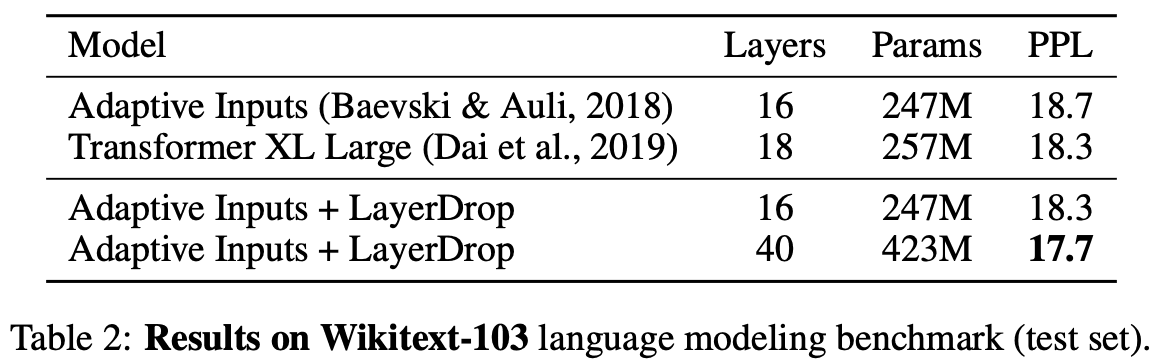Table 2 shows the experimental results for language modeling, showing the impact of LayerDrop on Transformers trained with Adaptive Inputs. Adding LayerDrop to a 16-layer Transformer improves the PPL by 0.4, which is consistent with the state-of-the-art results for Transformer XL. Adding LayerDrop to a 40-layer Transformer further improves the PPL by 0.6.

Very Deep transformers are unstable to train, use a lot of memory, are difficult to train, and are prone to overtraining on small datasets like Wikitext-103. LayerDrop normalizes the network and reduces memory usage, which increases the stability of training.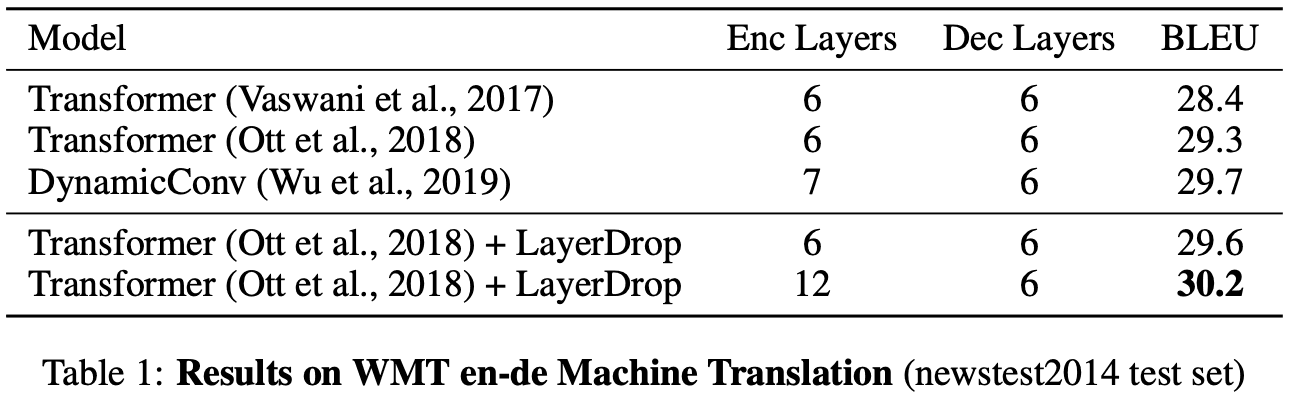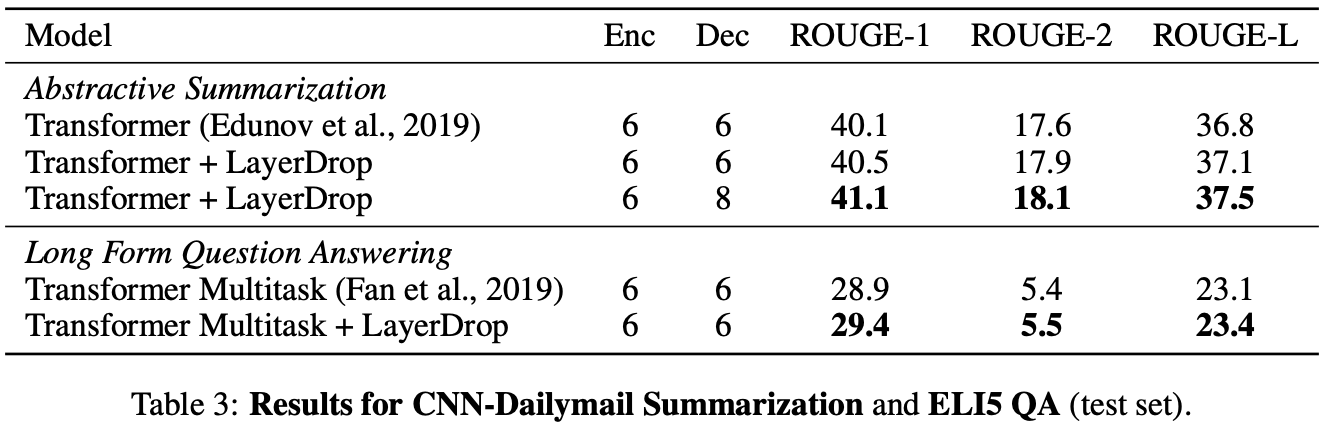Table 1 and Table 3 show the results for the Sequence to sequence task. We can see that applying LayerDrop to the models of the Machine Translation, Summarization, and Question and Answer tasks improve the performance of all tasks.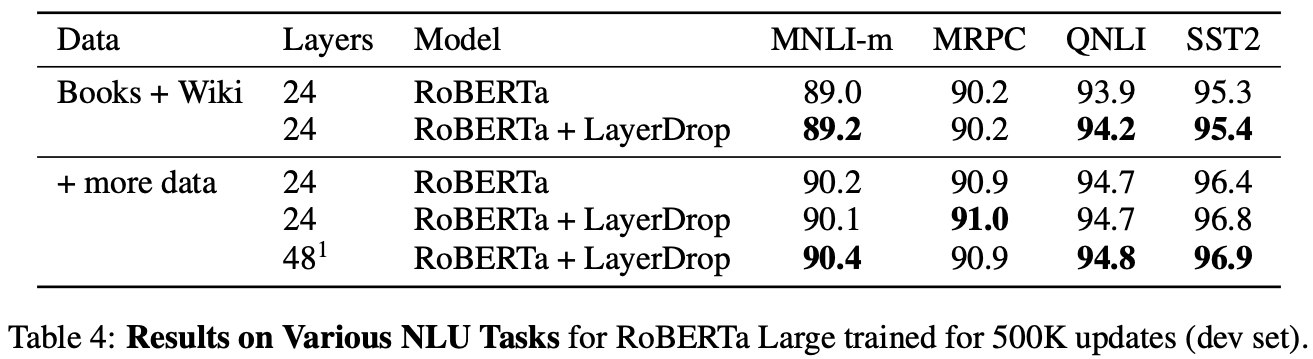Table 4 shows the results for pre-training. Similarly, we can see that LayerDrop improves performance.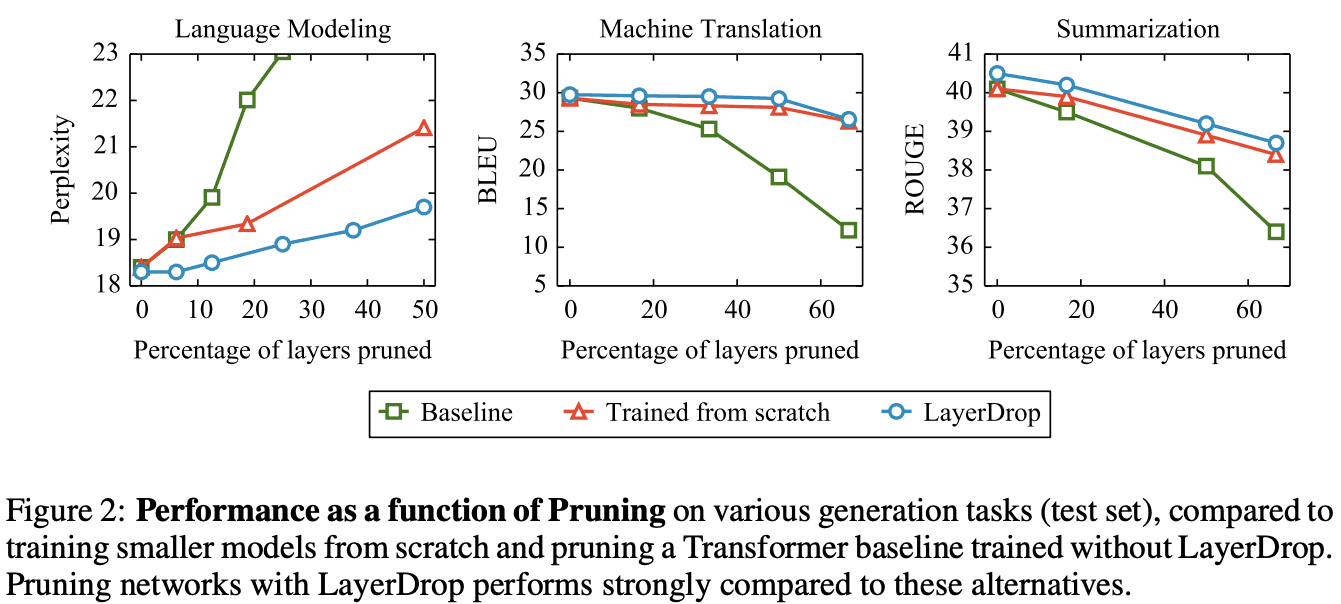Figure 2 shows the performance of the Transformer for language modeling, machine translation, and summarization, where the horizontal axis is the number of layers to be pruned and the vertical axis is the performance. On the other hand, it can be seen that the performance deteriorates significantly when layers are dropped from a baseline that does not use LayerDrop.

## Experiment ②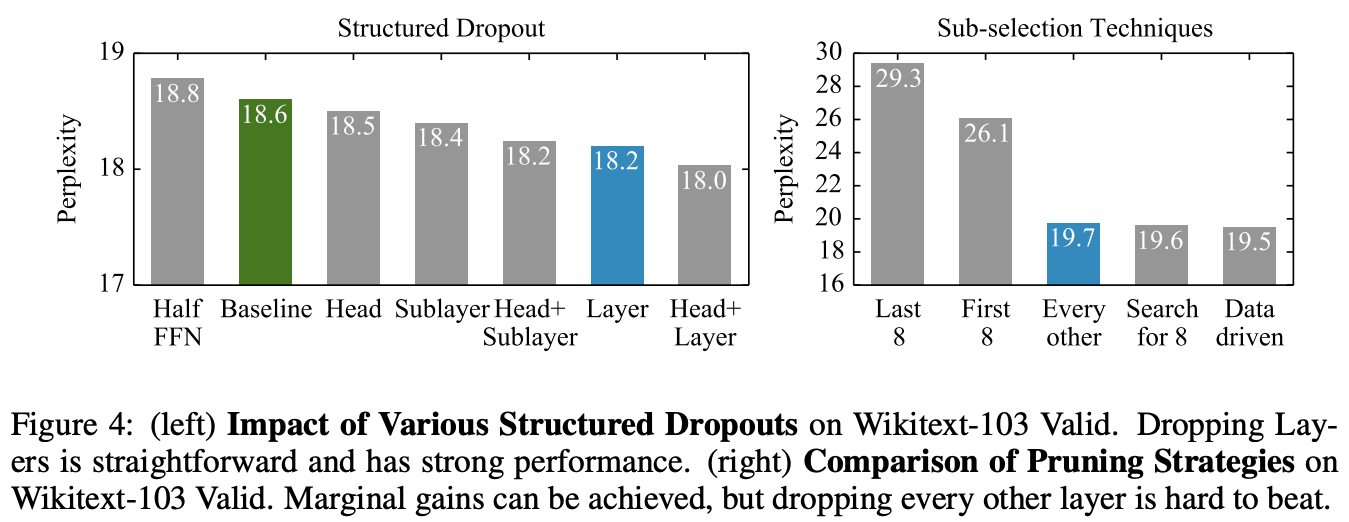The left panel of Figure 4 compares the dropout targets: the attention head, the FFN matrix, the whole transformer, and the results of a combination of each.

The right side of Figure 4 shows a comparison of approaches for selecting model layers during inference. In this paper, we use a simple method called "Every other", but it performs very well. Even the method that searches for the optimal 8 layers by learning shows only a small improvement compared to the proposed method. In contrast, it can be readily seen that dropping 8 layers from the input layer or 8 layers from the output layer results in a significant drop in performance. This is intuitive, but it is understandable that dropping the parts of the system that are processing inputs or predictions together will reduce performance.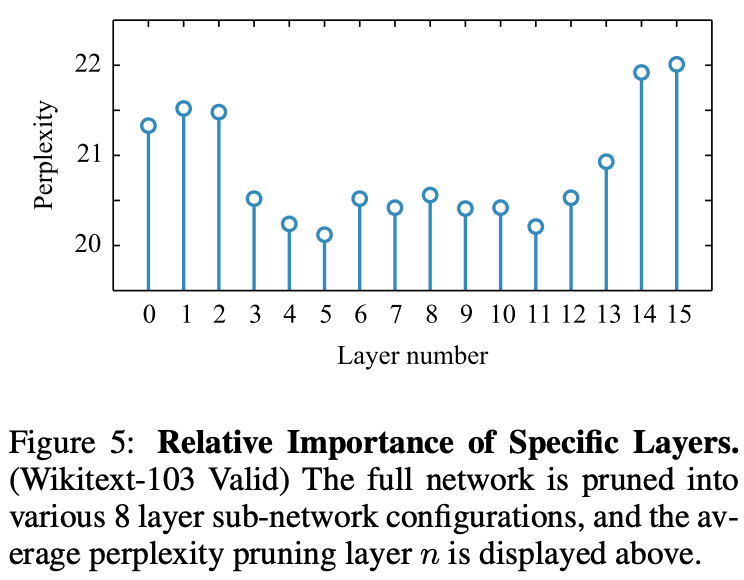Figure 5 shows the experimental results of which layers should be dropped. As you can see from the figure, the layers near the input layer and the output layer are the important layers (which should not be dropped).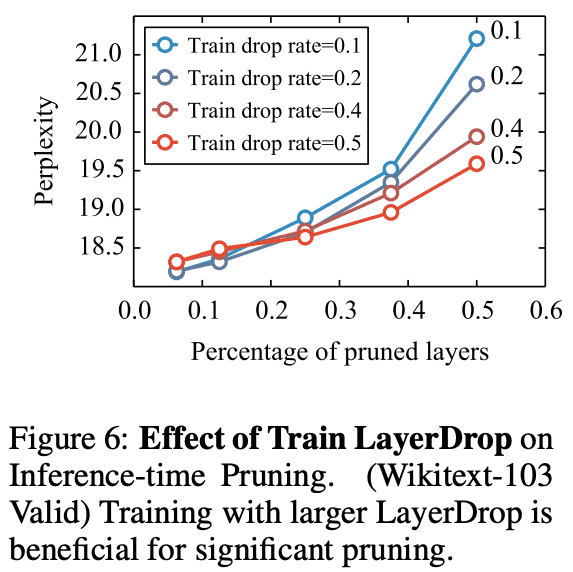Figure 6 shows the comparison of drop rate and performance. It can be seen that a higher drop rate is desirable for a deeper model.

## Summary

Dropout normalizes neural networks so that they become robust. In this paper, we proposed LayerDrop, which focuses on Layers in particular. We have shown that it can train very deep models for various text generation and machine translation tasks, stabilize them, and extract models of various depths with good performance.

If you have any suggestions for improvement of the content of the article,